# The currency drain ratio is 0.2 and the desired reserve ratio is 0.4. What is the...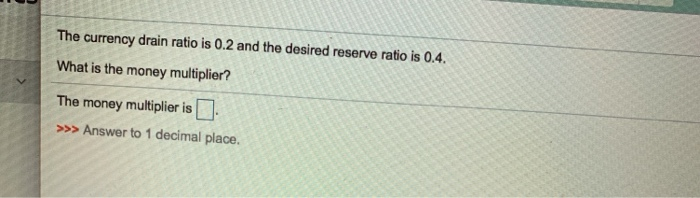The currency drain ratio is 0.2 and the desired reserve ratio is 0.4. What is the money multiplier? The money multiplier is >>> Answer to 1 decimal place.

Formula :

Money multiplier(m) = M/H

where M = Money supply = C + D where C = Currency, D = Deposit

H = Monetary Base = C + R where C = Currency, R = Desired reserves

=> Money multiplier(m) = M/H = (C + D)/C + R)

=> m = (C/D + 1)/(C/D + R/D)

where C/D = currency drain ratio = 0.2, R/D = Desired reserve ratio = 0.4

=> Money multiplier(m) = (C/D + 1)/(C/D + R/D) = (0.2 + 1)/(0.2 + 0.4) = 2

Hence, The money multiplier is 2.

^ You forgot the decimal place; its 0.02 & 0.04

= 17

##### Add Answer of: The currency drain ratio is 0.2 and the desired reserve ratio is 0.4. What is the...
Similar Homework Help Questions
• ### If the central bank prints \$5000, which is handed to citizens with a currency ratio of 0.2 and a bankers’ reserve ratio...

If the central bank prints \$5000, which is handed to citizens with a currency ratio of 0.2 and a bankers’ reserve ratio of 0.2, what will happen? What is the final chance to the money supply? What is the money multiplier?

• ### Compute the impact on the money multiplier of an increase in the currency-to-deposit ratio from 10...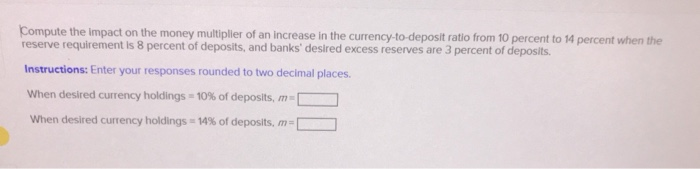Compute the impact on the money multiplier of an increase in the currency-to-deposit ratio from 10 percent to 14 percent when the reserve requirement Is 8 percent of deposits, and banks' desired excess reserves are 3 percent of deposits Instructions: Enter your responses rounded to two decimal places. When desired currency holdings 10 % of deposits, m When desired currency holdings 14 % of deposits, m Suppose the currency-to-deposit ratio is 0.2, the excess reserve-to-deposit ratio is 0.05, and the...

• ### 14) If the desired reserve ratio is ten percent, currency in circulation is \$400 billion, and...

14) If the desired reserve ratio is ten percent, currency in circulation is \$400 billion, and chequable deposits are \$800 billion, then the money multiplier is approximately ________. A) 2.5 B) 1.67 C) 2.0 D) 0.601 16) If the desired reserve ratio is ten percent, currency in circulation is \$400 billion, chequable deposits are \$800 billion, and excess reserves total \$0.8 billion, then the excess reserves-chequable deposit ratio is ________. A) 0.001 B) 0.10 C) 0.01 17) If the desired...

• ### Suppose the currency-to-deposit ratio is 0.25, the excess reserve-to-deposit ratio is 0.03, and the required reserve...

Suppose the currency-to-deposit ratio is 0.25, the excess reserve-to-deposit ratio is 0.03, and the required reserve ratio is 0.1. Which will have a larger impact on the money multiplier: a rise of 0.05 in the currency ratio or in the excess reserve ratio? Instructions: Enter your response rounded to two decimal places. If the currency-to-deposit ratio rises to 0.3, the multiplier will be m = If, instead, the excess reserve-to-deposit ratio rises, the multiplier will be m =

• ### The Fed buys \$3 million of securities from AIG. AlG has a desired reserve ratio of...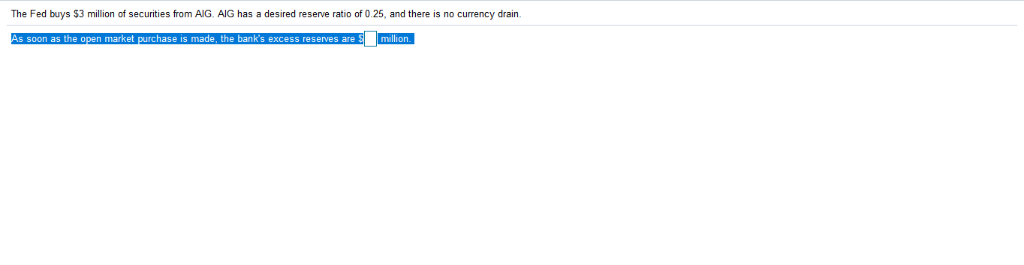The Fed buys \$3 million of securities from AIG. AlG has a desired reserve ratio of 0.25, and there is no currency drain purchase is made, the ban The Fed buys \$3 million of securities from AIG. AIG has a desired reserve ratio of 0.25, and there is no currency drain. As soon as the open market purchase is made, the bank's excess reserves are Smillion.

• ### Suppose the monetary base is \$100. If the currency-deposit ratio is 0.20 and the reserve-deposit ratio...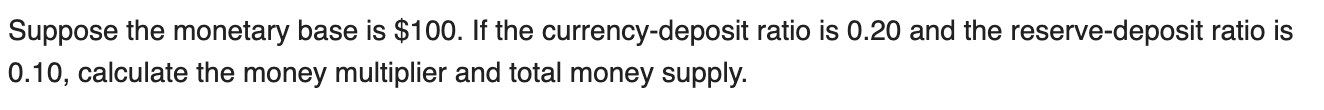Suppose the monetary base is \$100. If the currency-deposit ratio is 0.20 and the reserve-deposit ratio is 0.10, calculate the money multiplier and total money supply.

• ### Compute the impact on the money multiplier of an increase in the currency-to-deposit ratio from 10...

Compute the impact on the money multiplier of an increase in the currency-to-deposit ratio from 10 percent to 15 percent when the reserve requirement is 10 percent of deposits, and banks’ desired excess reserves are 3 percent of deposits. Instructions: Enter your responses rounded to two decimal places. When desired currency holdings = 10% of deposits, m = When desired currency holdings = 15% of deposits, m =

• ### Required reserve ratio is 0,2, there are 217B currency in circulation, 667B checkable deposits, and 4,6B...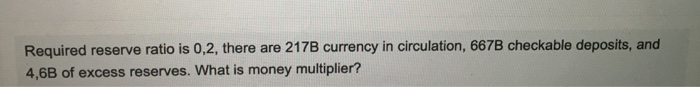Required reserve ratio is 0,2, there are 217B currency in circulation, 667B checkable deposits, and 4,6B of excess reserves. What is money multiplier?

• ### Suppose that r= required reserve ratio = 0.20 c = {C/D) = currency ratio = 0.30...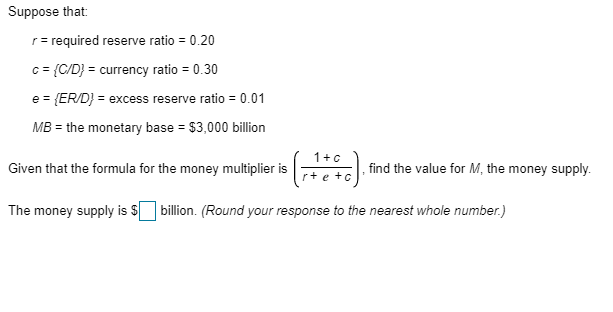Suppose that r= required reserve ratio = 0.20 c = {C/D) = currency ratio = 0.30 e = {ER/D} = excess reserve ratio = 0.01 MB = the monetary base = \$3,000 billion Given that the formula for the money multiplier is find the value for M, the money supply. The money supply is \$ billion. (Round your response to the nearest whole number.)

• ### Consider the following situations a. Bank reserves are \$3O0, the public holds \$200 in currency, and the desired rese...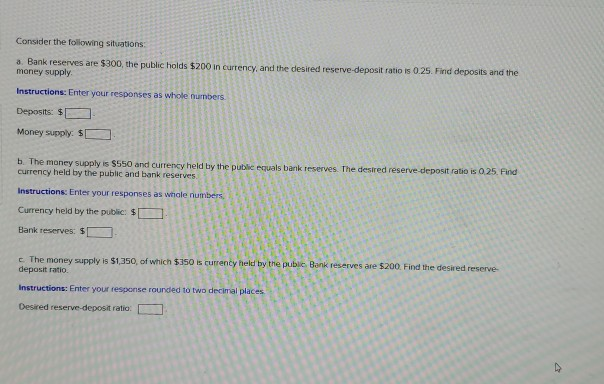Consider the following situations a. Bank reserves are \$3O0, the public holds \$200 in currency, and the desired reserve-deposit ratio is 0.25. Find deposits and the money supply Instructions: Enter your responses as whole numbers Deposits: \$ Money supply. \$ b. The maney supply is \$550 and currency held by the public equals bank reserves The desired reserve-deposit ratio is 025 Find currency held by the public and bank reserves. Instructions: Enter your responses as whale numbers Currency held by...

Free Homework App